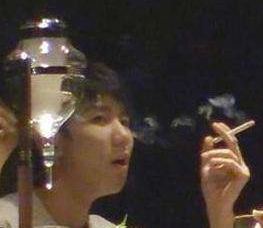TFBOYS组合成员、19岁的王源在北京“桐寿司”日本料理餐厅吃饭，席间他熟练叼起香烟吞云吐雾，网民沸腾了:一直是大家眼中的乖宝宝、富有满满正能量的二字弟弟怎么也会抽烟了呢？王源公开致歉：做了一个错误的示范

这段视频一出，#王源抽烟#一天N次上热搜，仅新浪微博阅读16.3亿，讨论41.7万，就连他的道歉都达到13.4亿，吃瓜群众扒皮出当初他接受采访说希望父亲戒烟的内容，认为王源之前说“爸爸有抽烟的习惯，他现在挺自觉的，都会到外面去抽”这句话格外讽刺，因为王源是在明令禁止室内吸烟的北京市被拍到室内吸烟……

一时间微博上关于这一事件的热搜竟有十几个！很快王源公开致歉了：对不起！这件事让我深刻反思了自己的行为，对自己造成的不良社会影响，我感到十分抱歉和愧疚，很抱歉我做了一个错误的示范，我会承担相应的责任并接受处罚。作为一名公众人物，我今后一定会更加注重自身的言行，希望大家不要效仿我的错误行为。再次向大家道歉，我一定会以此为鉴，成为更好的大人。

北京市控烟协会：公众人物应成为模范

北京市控烟协会会长张建枢说，王源作为公众人物、作为大家喜爱的青年偶像，在公众场合公开违反《北京市控制吸烟条例》规定的室内公共场所禁止吸烟，显然是不对的。“这件事不要看做是小事。”张建枢说，此事是公众人物是否守法的一个具体体现，公众人物应成为模范，而不是起到负面带头作用。

中国控制吸烟协会公益法律专业委员会秘书长李恩泽表示，目前全国在控烟立法方面尚没有统一，各地执法标准各异，是控烟面临的首要问题。立法亟须统一，执法尺度才能达到一致，控烟力度才可能切实得到推进。

北京市卫生监督所：已派人到现场调查取证

5月21日，北京市卫生监督所党委副书记王本进表示，朝阳区卫生监督所已派人到现场调查取证。王本进指出，按照法律规定，在公共场所吸烟，场所不劝阻的话将遭受罚款5000-10000元，对于吸烟的个人一般将处罚50元，拒不改正的将处罚200元。@北京卫生监督发文表示，《北京市控制吸烟条例》规定，公共场所、工作场所的室内区域以及公共交通工具内禁止吸烟。针对媒体曝光事件，我市属地卫生监督机构正在进行核实调查。如果情况属实，将依法进行处罚。北京市朝阳区卫监所：责令该餐厅限期整改

5月21日下午，北京市朝阳区卫监所对王源抽烟时就餐的餐厅进行执法检查。现场，朝阳区卫监所的执法人员检查发现，餐厅没有禁止吸烟的标识，也没有相应的禁止吸烟管理制度，违反了相关要求。朝阳卫监所责令该餐厅限期改正，整改期间如果仍有问题，将依法进行处理。对于吸烟者，朝阳区卫监所表示，将依法约谈王源或者委托人。“公众人物在公共场合吸烟，按照法律是要进行罚款，接下来将依据行政执法程序，锁定相关证据，按法律程序进行处罚。热议

“乖宝宝”突然抽烟

“榜样”人设碎一地

自出道以来，作为TFboys的成员，王源和王俊凯、易烊千玺在大众心里一直都是“正能量”的代表人物。这次消息爆出，网络舆论圈便炸了，开始激烈讨论着王源应不应该抽烟、王源抽烟行为该不该被原谅等等。一时间王源的粉丝小汤圆们分成两大派别，一边坚持为王源洗白，而另一边则表示伤心脱粉。很多粉丝表示接受不了粉了那么多年的王源弟弟竟然刚成年就开始抽烟了。有网友说，“从小看到大的孩子，老母亲眼中的乖宝宝”突然抽烟了，实在是沉重的暴击。也有网友表示，本来就不喜欢别人抽烟，而喜欢了很多年的阳光少年王源弟弟怎么可以也有这种行为呢？

粉丝们纷纷对王源的少年感人设崩了一事表示十分失望，认为抽烟虽然算不上犯罪，但是王源做出了抽烟这一行为，违背了他一直以来的“乖宝宝”形象。

许多妈妈粉对此无比生气，尤其是在看了视频之后发现王源抽烟十分熟练，俨然就是一位老烟民。

作为一名养成系偶像的王源，是在粉丝们的眼中看着一步一步成长的，王源在大家的心里一直都是阳光上进的好孩子。但王源抽烟这一行为表明了他也会做不那么美好的事，也会被社会所影响，使得粉丝们心中关于他的干净阳光、积极乐观形象破灭，随即粉丝们接二连三地宣告从此脱粉。有网民对王源的抽烟行为也表示十分愤怒，认为公众人物不应该在公众场合抽烟，这样不仅违反了法律,还会带坏粉丝，尤其是像王源这样一位作为中国青少年代表的偶像。综合澎湃新闻、《新京报》等

思考

流量艺人抽烟到底是不是问题？如果是普通人抽烟，媒体懒得拍，民众也没那么大热情去讨论。但换成流量艺人，不管是不是在禁烟区抽烟，都会成为大问题。尤其是王源这类童星出身的艺人，更一直被冠以阳光大男孩的人设，他所在组合的队友对于吸烟这个问题也向来是避而远之。队长王俊凯发过怼抽烟行为的微博，队友易烊千玺更是世界卫生组织中国控烟倡导者，两年前就拍摄过控烟广告，接受采访说吸烟不仅对自身危害大，更会因二手烟而影响到年龄更小的孩子们，为了青少年的成长，大家也应当严于律己。

在王源抽烟视频爆出之前，他也是类似的正面形象人设，而这段视频直接摧毁了这个人设。其实，明星人设的问题探讨，真的已经成为老生常谈。什么是人设？人设其实是一个社会各方揣着明白装糊涂的一场共谋。这是一种消费预期，经纪公司把这个人作为一种商品推出来，粉丝消费他不仅是消费他的歌、他的戏，还在消费他代表的价值观。这个人设可能是暖男，可能是阳光少年，可能是好丈夫，可能是好妈妈什么的，艺人会给大众一种期待，其实消费者可能也知道这个人设并不完全真实。消费者没有大众想象中那么单纯，可能会知道明星人设有公司包装的功劳，但是他们并不在意，因为这种人设是他们喜欢并想要消费的。反过来，明星一旦让粉丝的消费落空了，你就得承担后果，粉丝心里明不明白是一回事，但是你表现出来的符不符合人设又是另外一回事，你经营的这个人设让你收了多少好处，多少人因为这个喜欢你替你花钱，然后有一天你要接受同样东西的反噬。有人说中国没有偶像明星人设毕业的机制，要么你不红被人遗忘了，要么就这样崩塌当做你的毕业方式。也正是因为如此，更应该珍惜自己的人设。（责任编辑：士剑波）

• <<<<<<<<<
• <<<<<<<<<
• <<<<<<<<<
• <<<<<<<<<
• <<<<<<<<<
• <<<<<<<<<
• <<<<<<<<<
• <<<<<<<<<
• <<<<<<<<<
• <<<<<<<<<
• <<<<<<<<<
• <<<<<<<<<
• <<<<<<<<<
• <<<<<<<<<
• <<<<<<<<<
• <<<<<<<<<
• <<<<<<<<<
• <<<<<<<<<
• <<<<<<<<<
• <<<<<<<<<
• <<<<<<<<<
• <<<<<<<<<
• <<<<<<<<<
• <<<<<<<<<
• <<<<<<<<<
• <<<<<<<<<
• <<<<<<<<<
• <<<<<<<<<
• <<<<<<<<<
• <<<<<<<<<
• <<<<<<<<<
• <<<<<<<<<
• <<<<<<<<<
• <<<<<<<<<
• <<<<<<<<<
• <<<<<<<<<
• <<<<<<<<<

• <<<<<<<<<

# 商业资讯<<<<<<<<<

• [<<<<<<<<<] <<<<<<<<<
• [<<<<<<<<<] <<<<<<<<<
• [<<<<<<<<<] <<<<<<<<<
• [<<<<<<<<<] <<<<<<<<<
• [<<<<<<<<<] <<<<<<<<<
• [<<<<<<<<<] <<<<<<<<<
• [<<<<<<<<<] <<<<<<<<<
• [<<<<<<<<<] <<<<<<<<<
• [<<<<<<<<<] <<<<<<<<<
• [<<<<<<<<<] <<<<<<<<<
• [<<<<<<<<<] <<<<<<<<<
• [<<<<<<<<<] <<<<<<<<<